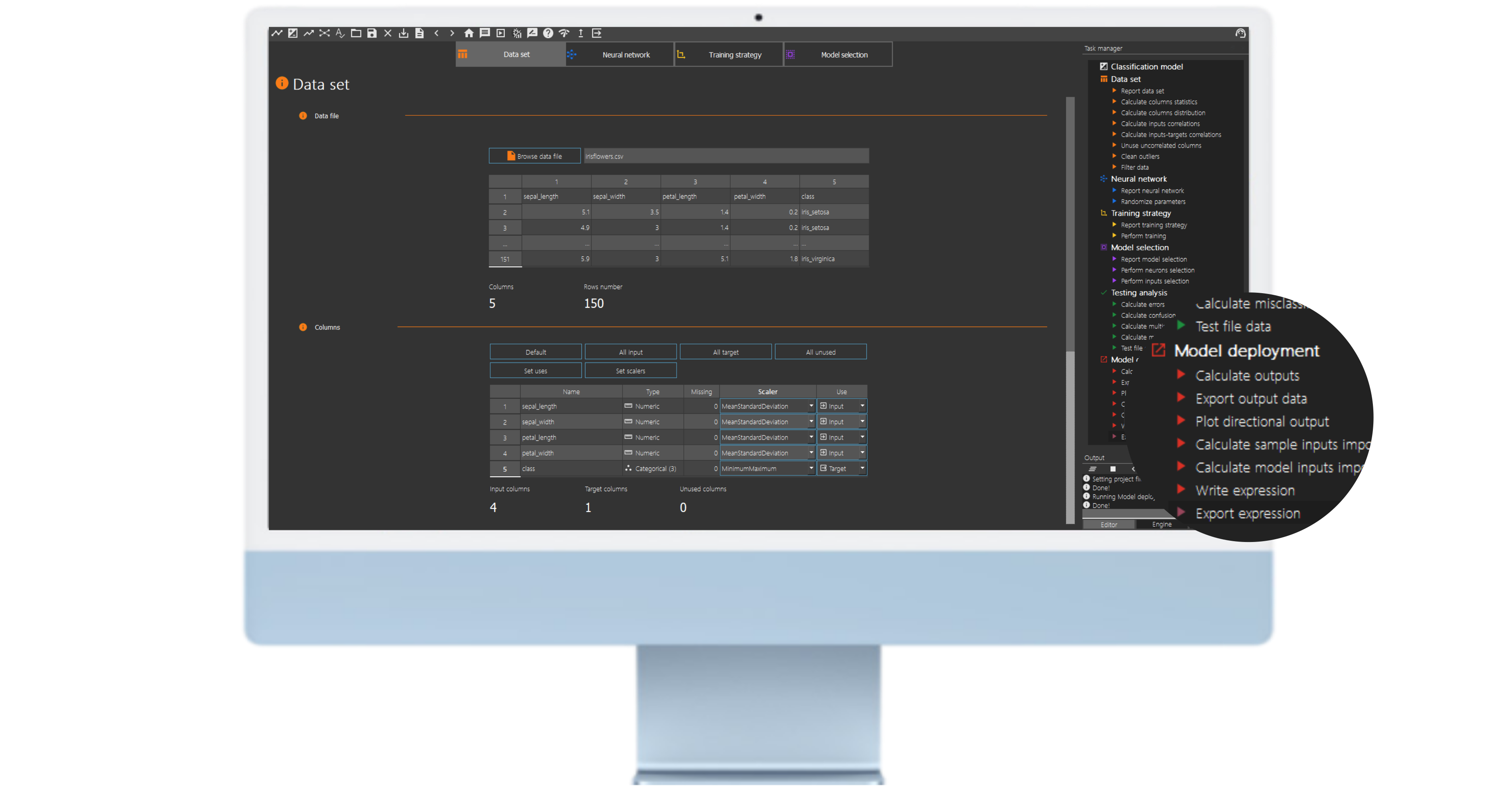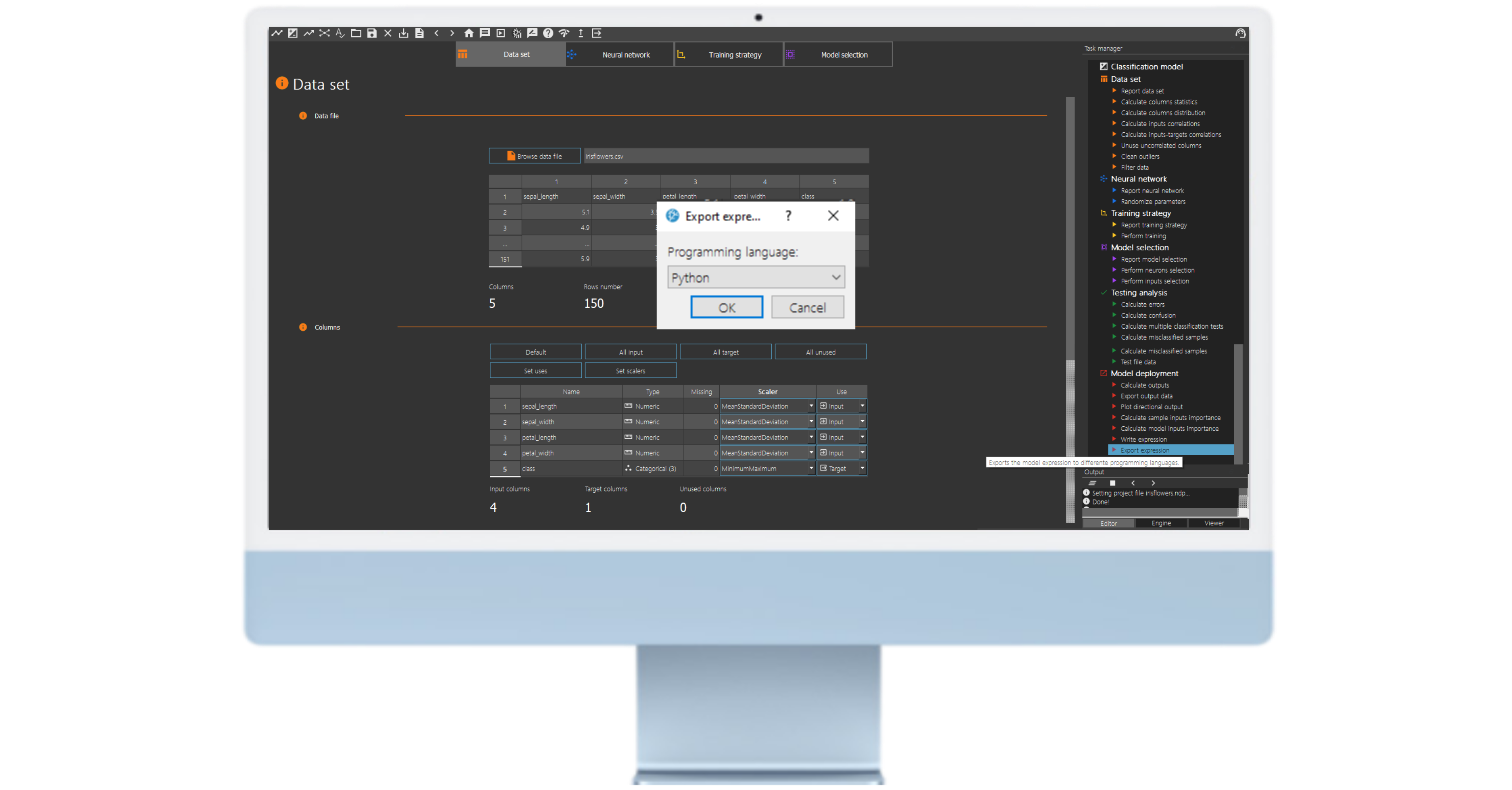## 1. Introduction

Neural Designer is a powerful tool for building and analyzing neural network models. However, when working with these models, it is crucial to have access to the underlying mathematical expressions that govern their behavior. Fortunately, Neural Designer provides several options for working with these expressions.

The predictive model takes the form of a function of the outputs concerning the inputs.

We can export the mathematical expression the model represents to different programming languages.

In this tutorial, we will explain how to export the mathematical expression of our model in the JS programming language.

## 2. How to export the mathematical expression?

Once we have trained the model and performed the testing analysis, the neural network can predict outputs for inputs it has never seen. This process is called model deployment.

We obtain the mathematical expression of the trained neural network.

We can implement this expression in any programming language to obtain the output for our input.

### Example: The mathematical expression of the iris flower classification model

Classification of iris flowers is the best-known example of machine learning.

The aim is to classify iris flowers among three species (Setosa, Versicolor, or Virginica) from the measurements of sepals’ and petals’ length and width.

The central goal is to design a model that makes proper classifications for new flowers. In other words, one which exhibits good generalization.

After training and testing the model, we move on to the model deployment process.One such option is “write expression”, which allows users to view the mathematical expression represented by the neural network of the model. By clicking on this option, Neural Designer will display the model’s function to predict outputs based on inputs. This information can be crucial for understanding how the model works and making modifications to improve its performance.

Another option available to users is “export expression”. Users can export the mathematical expression the model represents in several programming languages by selecting this option. This can be particularly useful for incorporating the model into other software programs or integrating it into larger systems.To export the mathematical expression in JS code, choose this option from the drop-down menu and save it in the model directory.

## 3. Using a Pre-Trained Neural Network Model in JS

Exporting the mathematical expression of a pre-trained neural network in JS allows us to use the trained model to make predictions on new data sets without the need to retrain the network from scratch.

### Example: Using the Pre-Trained iris flower classification model in JS

The next listing is iris flower classification model neural network in the JS programming language

 <!-- Artificial Intelligence Techniques SL artelnics@artelnics.com

Your model has been exported to this JavaScript file. You can manage it with the main method, where you can change the values of your inputs. For example:

if we want to add these 3 values (0.3, 2.5 and 1.8) to our 3 inputs (Input_1, Input_2 and Input_1), the main program has to look like this:

Inputs Names:<!--Artificial Intelligence Techniques SLartelnics@artelnics.com
int neuralNetwork(){vector<float> inputs(3);
const float asdas = 0.3;inputs = asdas;const float input2 = 2.5;inputs = input2;const float input3 = 1.8;inputs = input3;. . .
Inputs Names:0) sepal_length1) sepal_width2) petal_length3) petal_width-->
<!DOCTYPE HTML><html lang="en">
<style>.button{background-color: #6F8FAF;border: none; color: white;text-align: center;font-size: 16px;margin: 4px 4px;cursor: pointer;}</style>
<body>
<section><br/>
<div align="center" style="display:block;text-align: center;"><!-- MENU OPTIONS HERE --><form style="display: inline-block;margin-left: auto; margin-right: auto;">
<table border="1px" class="form">
INPUTS<!-- 0scaling layer --><tr style="height:3.5em"><td> sepal_length </td><td style="text-align:center"><input type="range" id="sepal_length" value="6.1" min="4.3" max="7.9" step="0.036" onchange="updateTextInput1(this.value, 'sepal_length_text')" /><input class="tabla" type="number" id="sepal_length_text" value="6.1" min="4.3" max="7.9" step="0.036" onchange="updateTextInput1(this.value, 'sepal_length')"></td></tr>
<!-- 1scaling layer --><tr style="height:3.5em"><td> sepal_width </td><td style="text-align:center"><input type="range" id="sepal_width" value="3.2" min="2" max="4.4" step="0.024" onchange="updateTextInput1(this.value, 'sepal_width_text')" /><input class="tabla" type="number" id="sepal_width_text" value="3.2" min="2" max="4.4" step="0.024" onchange="updateTextInput1(this.value, 'sepal_width')"></td></tr>
<!-- 2scaling layer --><tr style="height:3.5em"><td> petal_length </td><td style="text-align:center"><input type="range" id="petal_length" value="3.95" min="1" max="6.9" step="0.059" onchange="updateTextInput1(this.value, 'petal_length_text')" /><input class="tabla" type="number" id="petal_length_text" value="3.95" min="1" max="6.9" step="0.059" onchange="updateTextInput1(this.value, 'petal_length')"></td></tr>
<!-- 3scaling layer --><tr style="height:3.5em"><td> petal_width </td><td style="text-align:center"><input type="range" id="petal_width" value="1.3" min="0.1" max="2.5" step="0.024" onchange="updateTextInput1(this.value, 'petal_width_text')" /><input class="tabla" type="number" id="petal_width_text" value="1.3" min="0.1" max="2.5" step="0.024" onchange="updateTextInput1(this.value, 'petal_width')"></td></tr>
</table></form>
<div align="center"><!-- BUTTON HERE --><button class="btn" onclick="neuralNetwork()">calculate outputs</button></div>
<br/>
<table border="1px" class="form">OUTPUTS<tr style="height:3.5em"><td> iris_setosa </td><td><input style="text-align:right; padding-right:20px;" id="iris_setosa" value="" type="text" disabled/></td></tr>
<tr style="height:3.5em"><td> iris_versicolor </td><td><input style="text-align:right; padding-right:20px;" id="iris_versicolor" value="" type="text" disabled/></td></tr>
<tr style="height:3.5em"><td> iris_virginica </td><td><input style="text-align:right; padding-right:20px;" id="iris_virginica" value="" type="text" disabled/></td></tr>
</table>
</form></div>
</section>
<script>function neuralNetwork(){var inputs = [];var sepal_length = document.getElementById("sepal_length").value;inputs.push(sepal_length);var sepal_width = document.getElementById("sepal_width").value;inputs.push(sepal_width);var petal_length = document.getElementById("petal_length").value;inputs.push(petal_length);var petal_width = document.getElementById("petal_width").value;inputs.push(petal_width);
var outputs = calculate_outputs(inputs);var iris_setosa = document.getElementById("iris_setosa");iris_setosa.value = outputs.toFixed(4);var iris_versicolor = document.getElementById("iris_versicolor");iris_versicolor.value = outputs.toFixed(4);var iris_virginica = document.getElementById("iris_virginica");iris_virginica.value = outputs.toFixed(4);update_LSTM();}
function calculate_outputs(inputs){var sepal_length = +inputs;var sepal_width = +inputs;var petal_length = +inputs;var petal_width = +inputs;
var scaled_sepal_length = (sepal_length-5.843329906)/0.8280659914;var scaled_sepal_width = (sepal_width-3.053999901)/0.4335939884;var scaled_petal_length = (petal_length-3.758670092)/1.764420033;var scaled_petal_width = (petal_width-1.19867003)/0.7631610036;
var perceptron_layer_1_output_0 = Math.tanh( -0.454828 + (scaled_sepal_length*-0.702639) + (scaled_sepal_width*1.58777) + (scaled_petal_length*-1.55384) + (scaled_petal_width*-1.00021) );var perceptron_layer_1_output_1 = Math.tanh( -2.33981 + (scaled_sepal_length*3.26766) + (scaled_sepal_width*0.645213) + (scaled_petal_length*2.71958) + (scaled_petal_width*3.2668) );var perceptron_layer_1_output_2 = Math.tanh( -3.16773 + (scaled_sepal_length*-0.00059909) + (scaled_sepal_width*-0.664832) + (scaled_petal_length*2.84724) + (scaled_petal_width*1.88258) );
var probabilistic_layer_combinations_0 = -0.53698 +3.6124*perceptron_layer_1_output_0 -3.02915*perceptron_layer_1_output_1 -0.0966353*perceptron_layer_1_output_2 ;var probabilistic_layer_combinations_1 = 1.54913 -1.85394*perceptron_layer_1_output_0 +0.152214*perceptron_layer_1_output_1 -2.55223*perceptron_layer_1_output_2 ;var probabilistic_layer_combinations_2 = -1.03593 -1.78098*perceptron_layer_1_output_0 +2.85544*perceptron_layer_1_output_1 +2.74533*perceptron_layer_1_output_2 ;
var sum = Math.exp(probabilistic_layer_combinations_0) + Math.exp(probabilistic_layer_combinations_1) + Math.exp(probabilistic_layer_combinations_2);
var iris_setosa = Math.exp(probabilistic_layer_combinations_0)/sum;var iris_versicolor = Math.exp(probabilistic_layer_combinations_1)/sum;var iris_virginica = Math.exp(probabilistic_layer_combinations_2)/sum;
var out = [];out.push(iris_setosa);out.push(iris_versicolor);out.push(iris_virginica);
return out;}
function updateTextInput1(val, id){document.getElementById(id).value = val;}
window.onresize = showDiv;
</script>
<!--script src="https://www.neuraldesigner.com/app/htmlparts/footer.js"></script-->
</body>
    </html>

The code you have shared is an HTML page with embedded JavaScript code. This web page allows interaction with a neural network model that has been exported from the Neural Designer software.

The JavaScript code is responsible for receiving input values from the sliders and text fields in the “INPUTS” section. Then, it uses those values to calculate the outputs of the neural network model using the calculate_outputs function. These outputs are displayed in the text fields in the “OUTPUTS” section.

To export this neural network model to your JavaScript project, you need to follow these steps:

1. Save the HTML and JavaScript code into a file with the “.html” extension, for example, “neural_network.html”.

2. Create an HTML page in your JavaScript project where you want to use the neural network model.

3. Inside your project’s HTML page, add an <iframe> tag to load the “neural_network.html” page that contains the exported model. For example:

 <iframe src="neural_network.html"></iframe>

Make sure the “neural_network.html” file is located in the same location as your project’s HTML page.

By loading the “neural_network.html” page in your JavaScript project, you will be able to interact with the neural network model and use it in your application. The input values can be modified using the sliders and text fields, and the outputs will be displayed in the corresponding text fields.

Remember that for the neural network model to work correctly, it’s important to load all the necessary dependencies and CSS styles, as done in the provided code.

## 4. Conclusions

The mathematical expression of a pre-trained neural network exported in different programming codes enables us to use the trained model to make predictions on new data sets without retraining the network from scratch.

Exporting the pre-trained mathematical expression of a neural network allows us to leverage the knowledge and generalization capability of the model in different applications and projects.
This is a fundamental step to take full advantage of the potential of artificial intelligence and machine learning in JS.